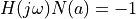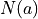# control.describing_function_plot¶

control.describing_function_plot(H, F, A, omega=None, refine=True, label='%5.2g @ %-5.2g', warn=None, **kwargs)[source]

Plot a Nyquist plot with a describing function for a nonlinear system.

This function generates a Nyquist plot for a closed loop system consisting of a linear system with a static nonlinear function in the feedback path.

Parameters
• H (LTI system) – Linear time-invariant (LTI) system (state space, transfer function, or FRD)

• F (static nonlinear function) – A static nonlinearity, either a scalar function or a single-input, single-output, static input/output system.

• A (list) – List of amplitudes to be used for the describing function plot.

• omega (list, optional) – List of frequencies to be used for the linear system Nyquist curve.

• label (str, optional) – Formatting string used to label intersection points on the Nyquist plot. Defaults to “%5.2g @ %-5.2g”. Set to None to omit labels.

• warn (bool, optional) – Set to True to turn on warnings generated by nyquist_plot or False to turn off warnings. If not set (or set to None), warnings are turned off if omega is specified, otherwise they are turned on.

Returns

intersections – A list of all amplitudes and frequencies in which, whereis the describing function associated with F, or None if there are no such points. Each pair represents a potential limit cycle for the closed loop system with amplitude given by the first value of the tuple and frequency given by the second value.

Return type

1D array of 2-tuples or None

Examples

>>> H_simple = ct.tf(, [1, 2, 2, 1])
>>> F_saturation = ct.saturation_nonlinearity(1)
>>> amp = np.linspace(1, 4, 10)
>>> ct.describing_function_plot(H_simple, F_saturation, amp)
[(3.343844998258643, 1.4142293090899216)]